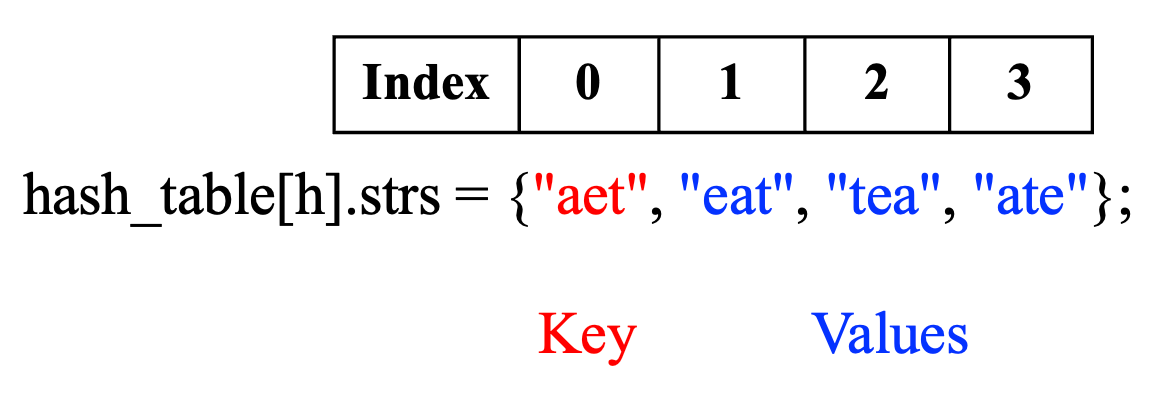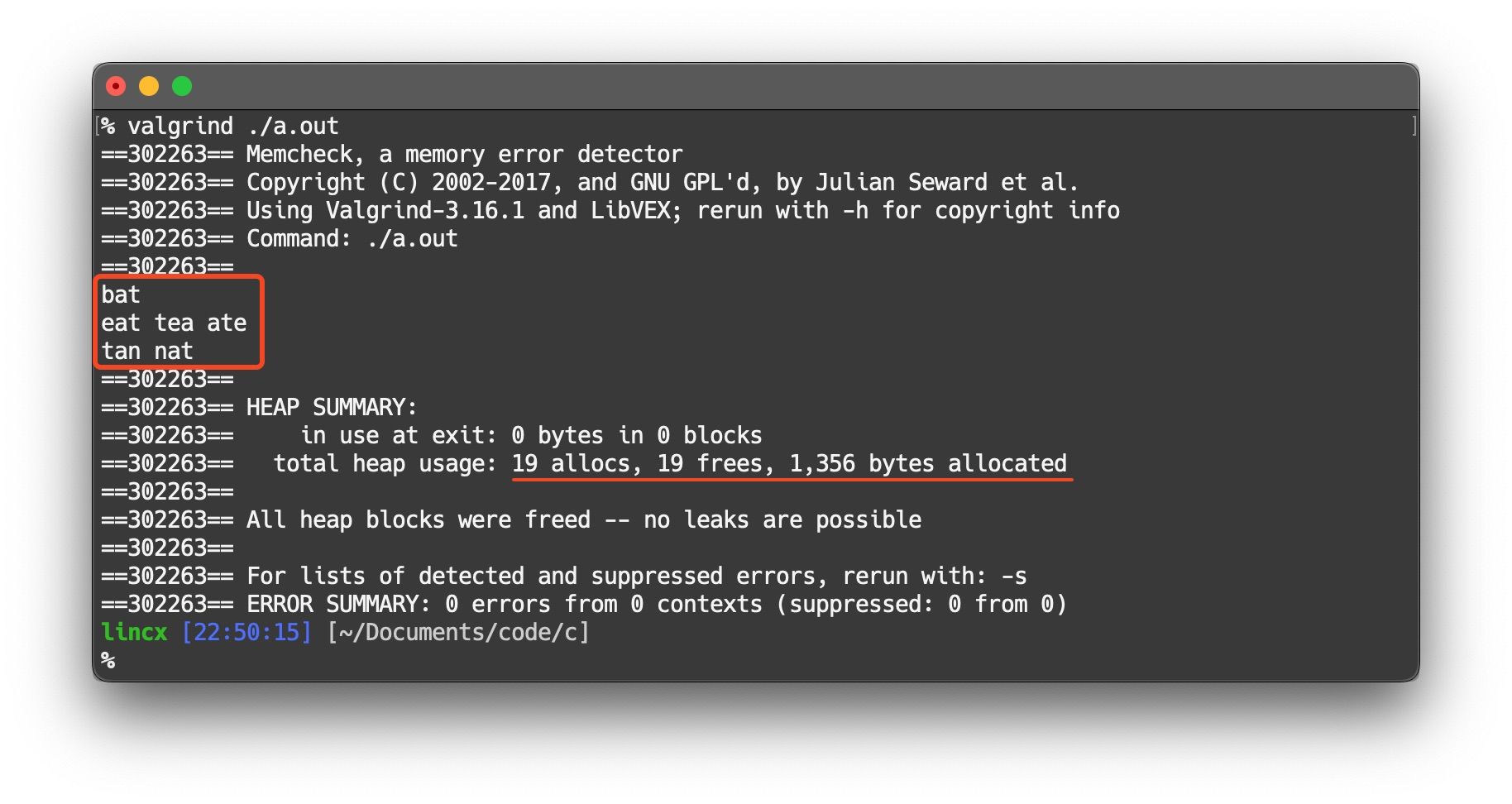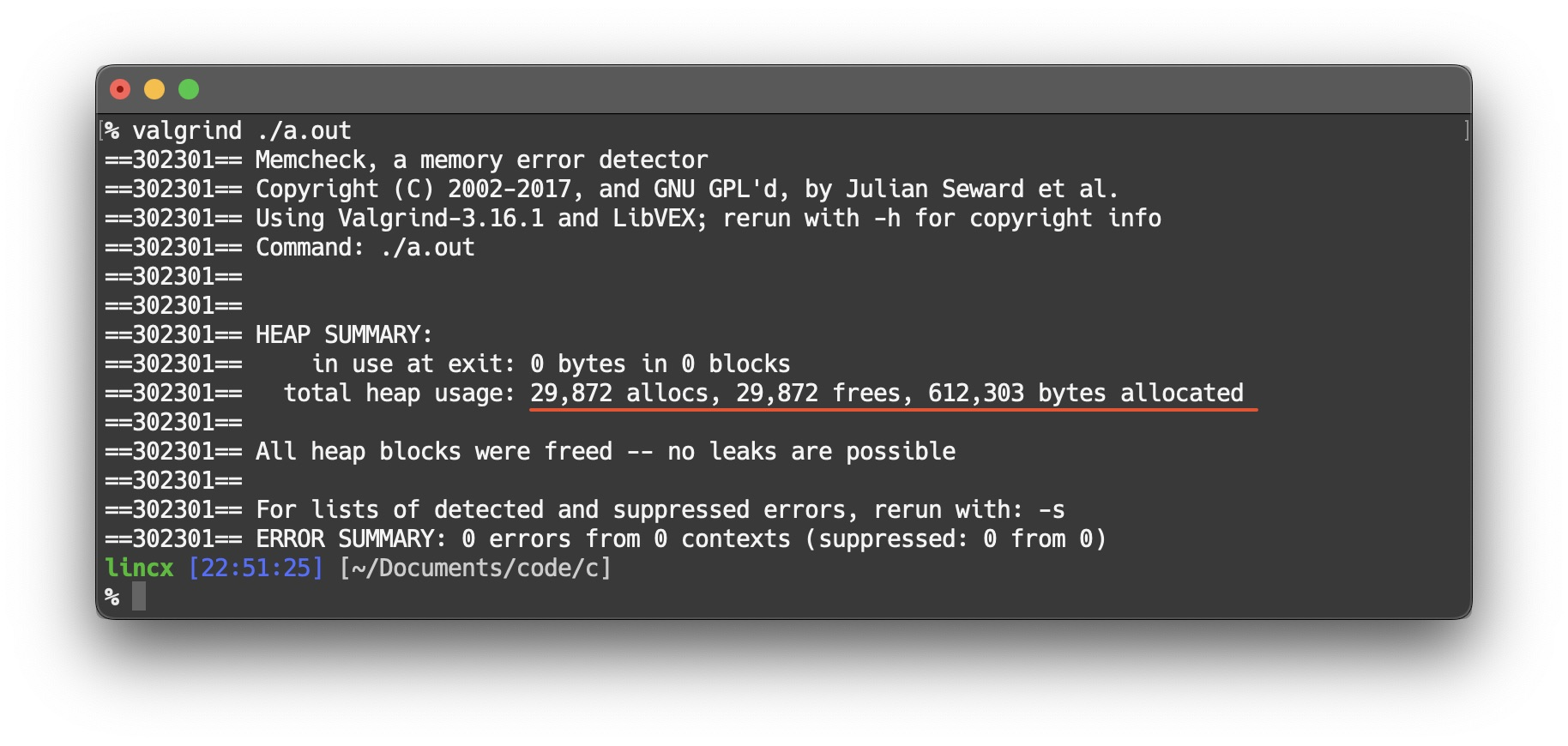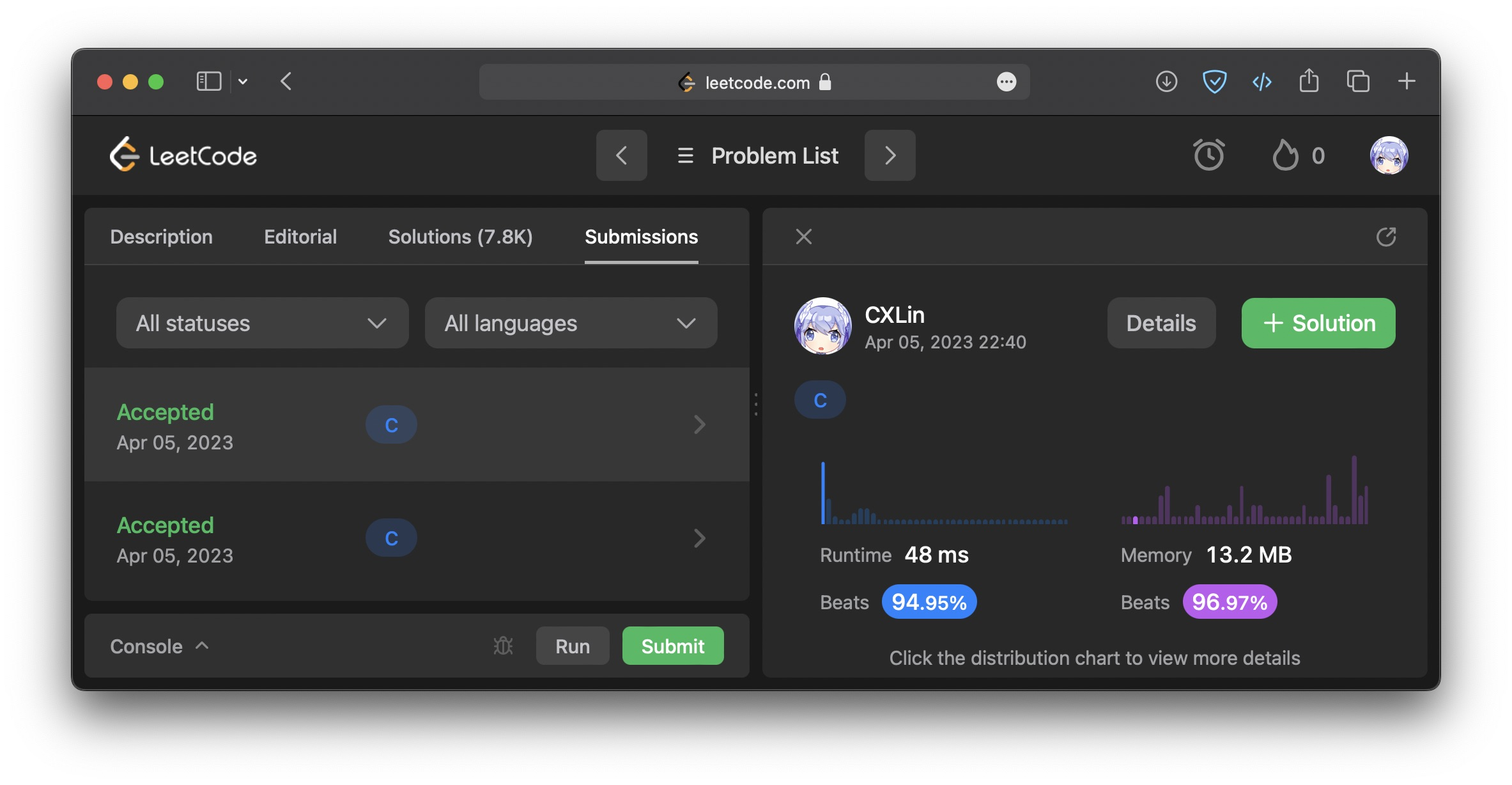LeetCode Problem 49, titled "Group Anagrams," asks to group a given set of strings based on their anagrams. Since the C language doesn't have the built-in hash table, solving this problem using C can be quite complex (painful). This article documents my C solution for Group Anagrams problem. I also provide complete code that can be run directly.

Anagrams are words formed by rearranging the letters of another word, using all the original letters exactly once. In Group Anagrams problem, the input is a list of strings, and the output should be a nested list where each group contains anagrams of each other.

The idea to solve this problem is to group anagrams together by storing the sorted input strings as keys, and the actual strings as values in a vector. After processing the entire string array, the hash table should be converted into a vector of string vectors as the answer. Therefore, this problem can be conveniently solved using C++, Java, Python, etc. Here is a C++ solution.

``````class Solution {
public:
vector<vector<string>> groupAnagrams(vector<string>& strs) {
unordered_map<string, vector<string>> anagram_map;

// use sorted str as key
for(const string &str : strs) {
string sorted_str = str;
sort(sorted_str.begin(), sorted_str.end());
anagram_map[sorted_str].push_back(str);
}

vector<vector<string>> ans;
for(const auto &entry : anagram_map) {
ans.push_back(entry.second);
}

return ans;
}
};``````

However, since the C language does not have a hash table, the main challenge of solving this problem by C is how to implement a hash table. Therefore, we need

• a struct that stores anagrams,
• a hashing algorithm that maps the string to a hash value,
• a function that adds a string to the hash bucket,
• and the `groupAnagrams()` function.

## String array struct and hashing algorithm

``````typedef struct {
char **strs;
int size;
int capacity;
} StrArr;

// BKDRHash
unsigned long BKDRHash(char *str) {
unsigned long h = 0;
for(; *str; ++str) {
h = h * 31 + *str;
}
return h;
}``````

Struct `StrArr` is equivalent to the hash bucket, which consists of a string array and two int data members. The string array `strs` stores the values (i.e., anagrams) of this bucket, `int size` counts the number of anagrams in `strs`, and `int capacity` records the allocated capacity, i.e., how many strings it can hold.

BKDRHash is utilized as the hashing algorithm, which is a simple and effective string hashing algorithm that maps a string to a hash value. The BKDRHash algorithm works by iterating through each character in a string and multiplying the current hash value by a large prime number, and then adding the ASCII value of the current character.

## Add a string to the hash bucket

``````// add a str to a hash bucket
void addStr(StrArr *arr, char *str) {
if(arr->size == arr->capacity) {
arr->capacity = (arr->capacity == 0) ? 1 : arr->capacity * 2;
arr->strs = (char **)realloc(arr->strs, arr->capacity * sizeof(char *));
}
arr->strs[arr->size++] = str;
}``````

`addStr()` receives a hash bucket and a string, and adds the string to this hash bucket. Before adding a new element, `addStr()` checks if the capacity is sufficient. If the capacity has been used up, it doubles the memory. Then, `addStr()` stores the address of the string.

## Group Anagrams

``````char ***groupAnagrams(char **strs, int strsSize, int *returnSize, int **returnColumnSizes) {
char ***ans = (char ***)malloc(strsSize * sizeof(char **));
*returnColumnSizes = (int *)malloc(strsSize * sizeof(int));
*returnSize = 0;

// take the sorted str as key, and the unsorted str as value
StrArr *hash_table = (StrArr *)calloc(strsSize, sizeof(StrArr));

// ...
}``````

The `ans` is the answer to be returned. The `groupAnagrams()` function first allocates the memory for answer and its meta data, initialize them, and create a hash table. The hash table has `sorted_str` as the key and `strs[i]` as the value. As shown below, `hash_table[h].strs` stores both the key and values: the first element of a hash bucket is the key, followed by the values.Hash table's structure.

Next, add `strs[i]` to the hash table iteratively, as follows.

``````char ***groupAnagrams(char **strs, int strsSize, int *returnSize, int **returnColumnSizes) {

// ...

for(int i = 0; i < strsSize; ++i) {
// compute hash value
char *sorted_str = strdup(strs[i]);
qsort(sorted_str, strlen(sorted_str), sizeof(char), cmp);
int h = BKDRHash(sorted_str) % strsSize;

// check for hash collisions
while(hash_table[h].size != 0 && strcmp(sorted_str, hash_table[h].strs) != 0) {
h = (h + 1) % strsSize;
}
// hash bucket's structure: hash_table[i] is the key, followed by the value
// the keys are stored in allocated memory (using `strdup`)
// while the values are already exist and created in main()
// therefore, only the key's memory need to be freed
if(hash_table[h].size == 0) {
}
else {
free(sorted_str);
}
}

// ...
}``````

`groupAnagrams()` first compute `strs[i]`'s hash value, which is obtained according to the sorted `strs[i]`. This ensures the hash value of the anagram is same. To fast access to h-th hash bucket, the hash value should to be smaller than the size of the given string array, i.e. 0 ≤ hash(sorted_str) < strsSize. Therefore, modulo is applied to the hash value, which may lead to hash collisions. To prevent different anagrams from being assigned to a same bucket, the hash value has to be checked first for collisions. If a bucket is non-empty and `sorted_str` is different from the first element (i.e., the key), the hash value should be incremented to avoid collisions. Then, add `strs[i]` to the correct hash bucket using `addStr()`. If this bucket is empty, the key should be added first. Note that only keys are stored in the allocated memory, while the values come from the input `strs[i]`. Therefore, only the first element of `hash_table[i].str` need to be deallocated to prevent memory leaks.

Next, `ans` stores the address of the hash table's values as the answer, as follows.

``````char ***groupAnagrams(char **strs, int strsSize, int *returnSize, int **returnColumnSizes) {

// ...

for(int i = 0; i < strsSize; ++i) {
if(hash_table[i].size > 0) {
// skip the key and return the value
ans[*returnSize] = hash_table[i].strs + 1;
(*returnColumnSizes)[*returnSize] = hash_table[i].size - 1;
++(*returnSize);
}
}
free(hash_table);
return ans;
}``````

Finally, deallocate the memory of hash table and return the answer.

The complete code for `groupAnagrams()` is shown bellow.

``````char ***groupAnagrams(char **strs, int strsSize, int *returnSize, int **returnColumnSizes) {
char ***ans = (char ***)malloc(strsSize * sizeof(char **));
*returnColumnSizes = (int *)malloc(strsSize * sizeof(int));
*returnSize = 0;

// take the sorted str as key, and the unsorted str as value
StrArr *hash_table = (StrArr *)calloc(strsSize, sizeof(StrArr));

for(int i = 0; i < strsSize; ++i) {
// compute hash value
char *sorted_str = strdup(strs[i]);
qsort(sorted_str, strlen(sorted_str), sizeof(char), cmp);
int h = BKDRHash(sorted_str) % strsSize;

// check for hash collisions
while(hash_table[h].size != 0 && strcmp(sorted_str, hash_table[h].strs) != 0) {
h = (h + 1) % strsSize;
}
// hash bucket's structure: hash_table[i] is the key, followed by the value
// the keys are stored in allocated memory (using `strdup`)
// while the values are already exist and created in main()
// therefore, only the key's memory need to be freed
if(hash_table[h].size == 0) {
}
else {
free(sorted_str);
}
}

for(int i = 0; i < strsSize; ++i) {
if(hash_table[i].size > 0) {
// skip the key and return the value
ans[*returnSize] = hash_table[i].strs + 1;
(*returnColumnSizes)[*returnSize] = hash_table[i].size - 1;
++(*returnSize);
}
}

free(hash_table);

return ans;
}``````

## Memory management

Although the content up to the previous section is already sufficient as a LeetCode solution, I usually make sure to free all the allocated memory to practice memory management skills in C. Valgrind is a tool suite for memory analysis, and it can be used to check for memory leaks. Since `hash_table` is not returned to `main()`, it should be deallocated in `groupAnagrams()`. Furthermore, only the key of each hash bucket needs to be freed. Since the key is located at `hash_table[i].strs`, and `ans[*returnSize] = hash_table[i].strs`, the key can be found at `(ans[i] - 1)`. Thus, memory can be reclaimed in the `main()` function as follows.

``````int main() {
// ...

// free the memory
for(int i = 0; i < *returnSize; ++i) {
// free the first str, i.e., the key of this hash bucket
free((ans[i] - 1));
// free the string array
free(ans[i] - 1);
}
free(ans);
free(returnColumnSizes);
free(returnSize);
return 0;
}``````

## Demo and memory analysis

The following image shows the result when strs = {"eat", "tea", "tan", "ate", "nat", "bat"}.The result when strsSize = 10,000.All allocated memory was freed.

Beats 95% in time and 97% in space.Outline